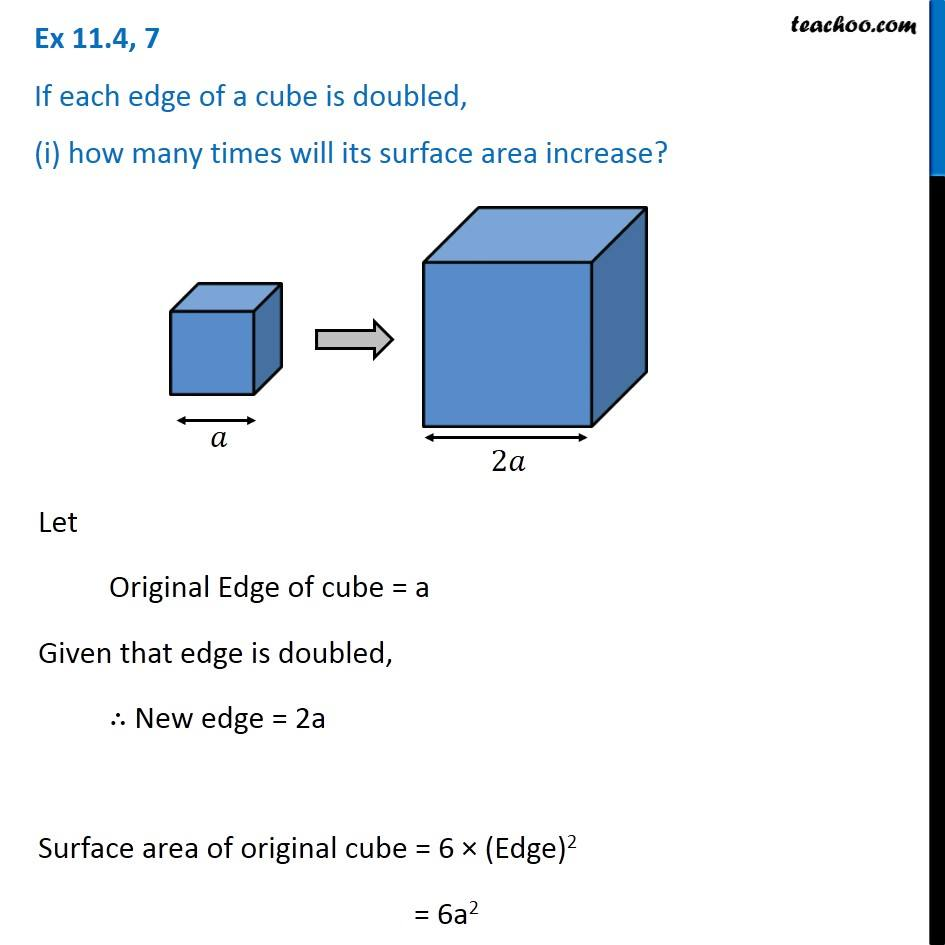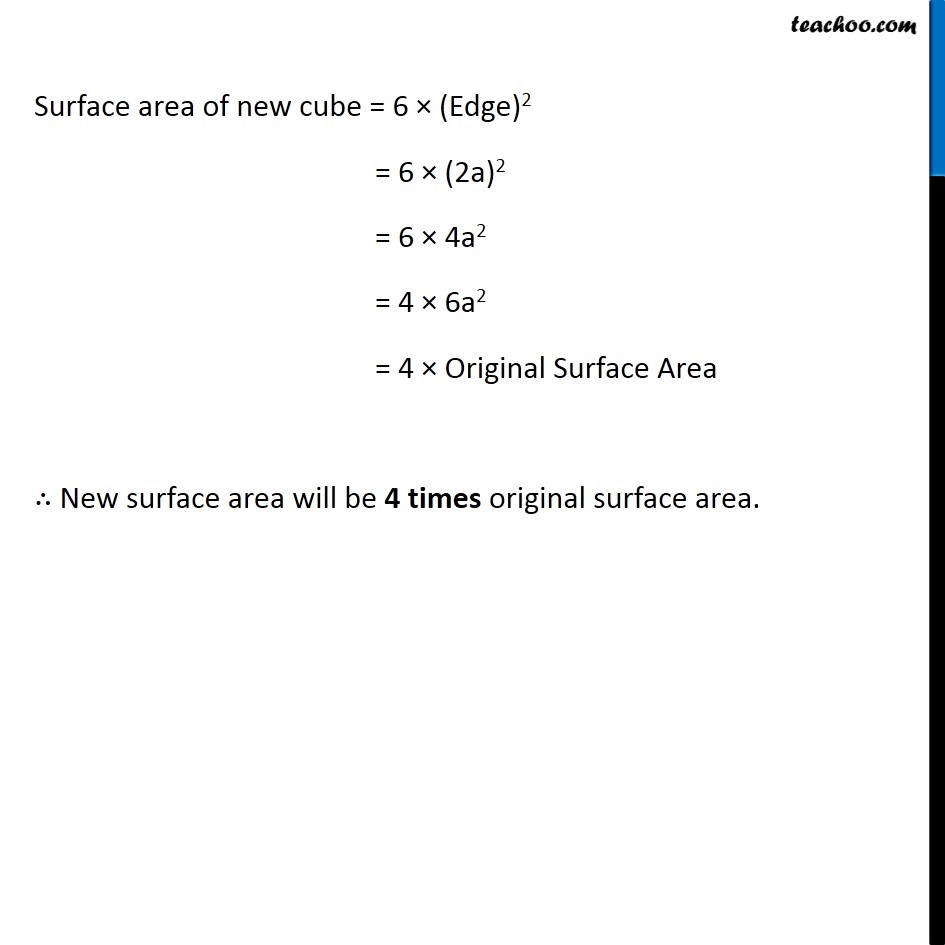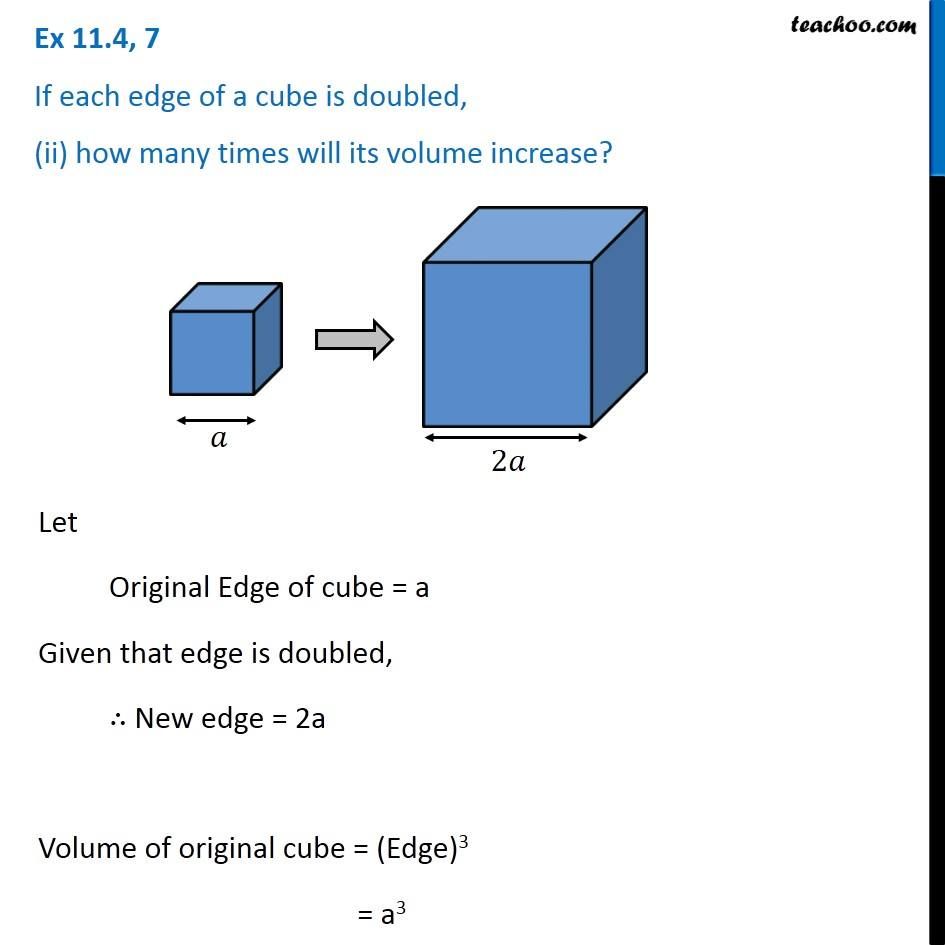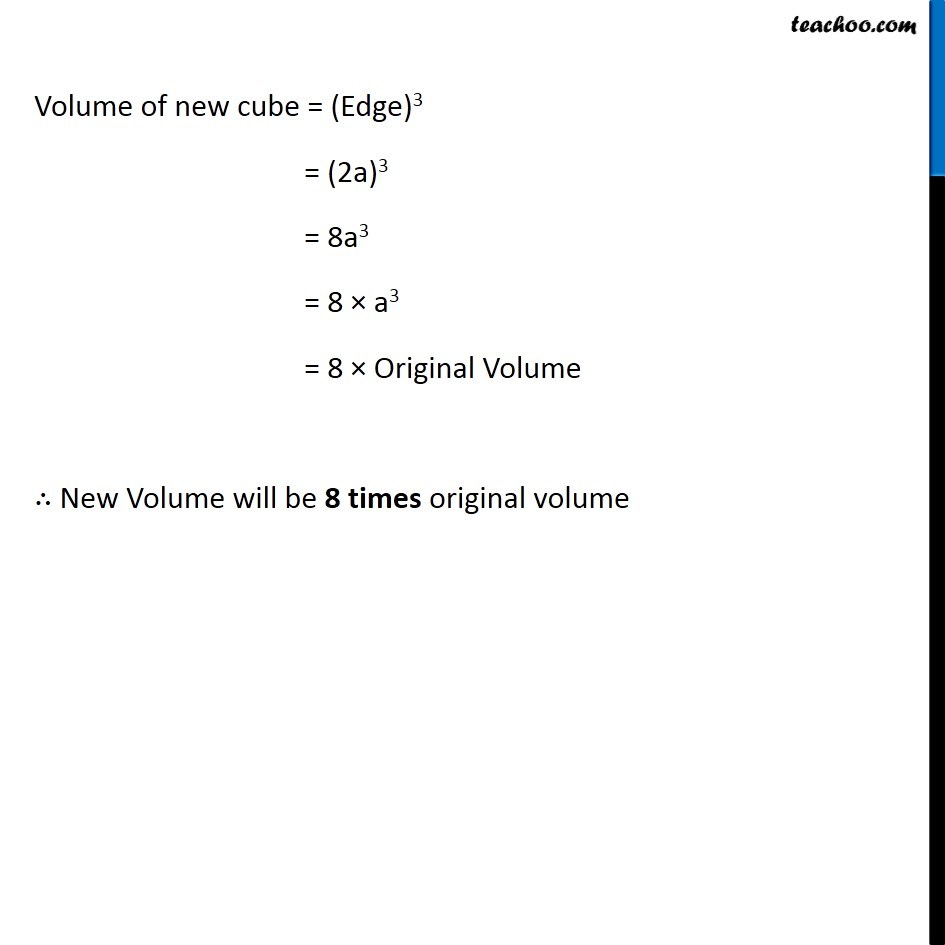Subscribe to our Youtube Channel - https://you.tube/teachoo

1. Chapter 11 Class 8 Mensuration
2. Serial order wise
3. Ex 11.4

Transcript

Ex 11.4, 7 If each edge of a cube is doubled, (i) how many times will its surface area increase? Let Original Edge of cube = a Given that edge is doubled, ∴ New edge = 2a Surface area of original cube = 6 × (Edge)2 = 6a2 Surface area of new cube = 6 × (Edge)2 = 6 × (2a)2 = 6 × 4a2 = 4 × 6a2 = 4 × Original Surface Area ∴ New surface area will be 4 times original surface area. Surface area of new cube = 6 × (Edge)2 = 6 × (2a)2 = 6 × 4a2 = 4 × 6a2 = 4 × Original Surface Area ∴ New surface area will be 4 times original surface area. Ex 11.4, 7 If each edge of a cube is doubled, (ii) how many times will its volume increase? Let Original Edge of cube = a Given that edge is doubled, ∴ New edge = 2a Volume of original cube = (Edge)3 = a3 Surface area of new cube = 6 × (Edge)2 = 6 × (2a)2 = 6 × 4a2 = 4 × 6a2 = 4 × Original Surface Area ∴ New surface area will be 4 times original surface area. Volume of new cube = (Edge)3 = (2a)3 = 8a3 = 8 × a3 = 8 × Original Volume ∴ New Volume will be 8 times original volume

Ex 11.4

Chapter 11 Class 8 Mensuration
Serial order wise

About the AuthorDavneet Singh
Davneet Singh is a graduate from Indian Institute of Technology, Kanpur. He has been teaching from the past 10 years. He provides courses for Maths and Science at Teachoo.